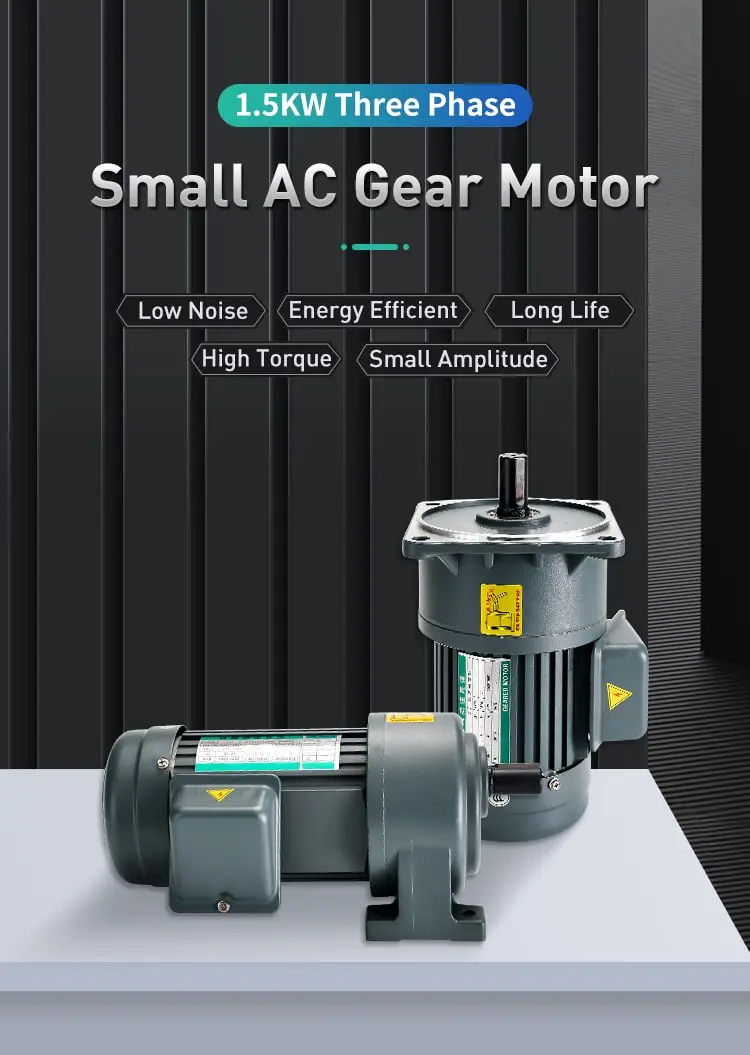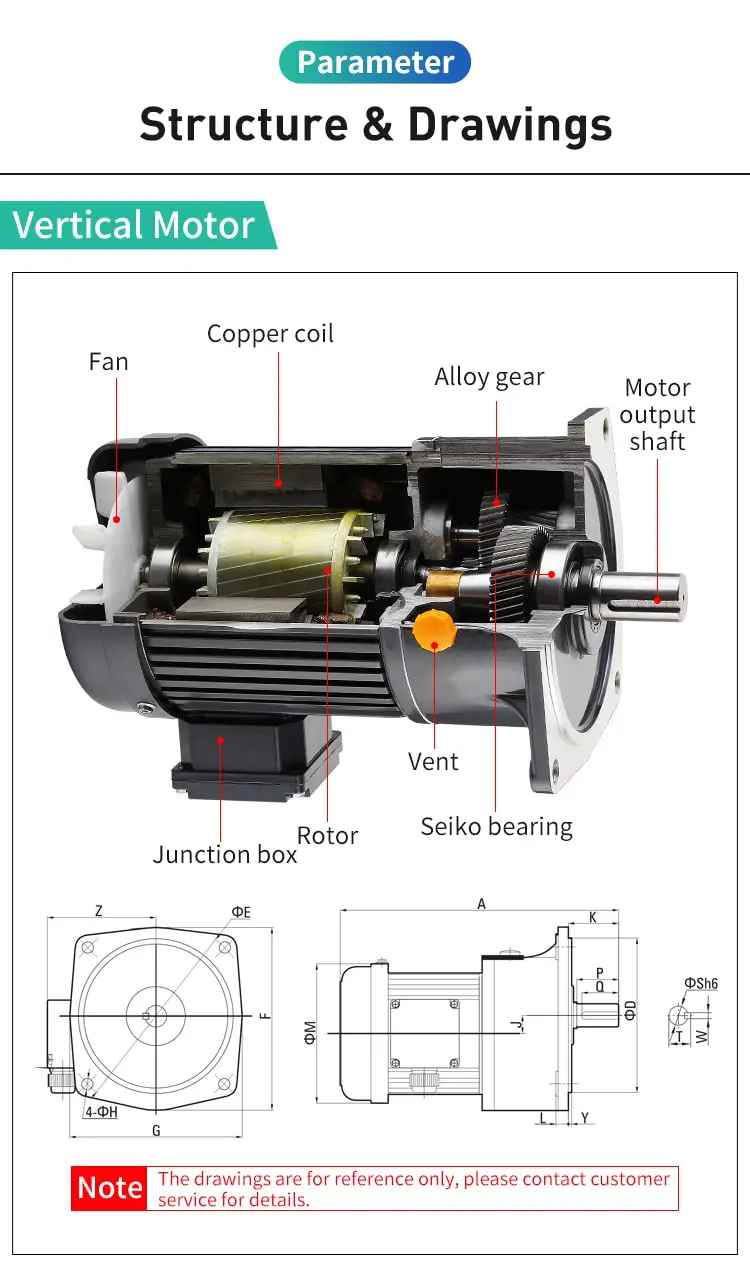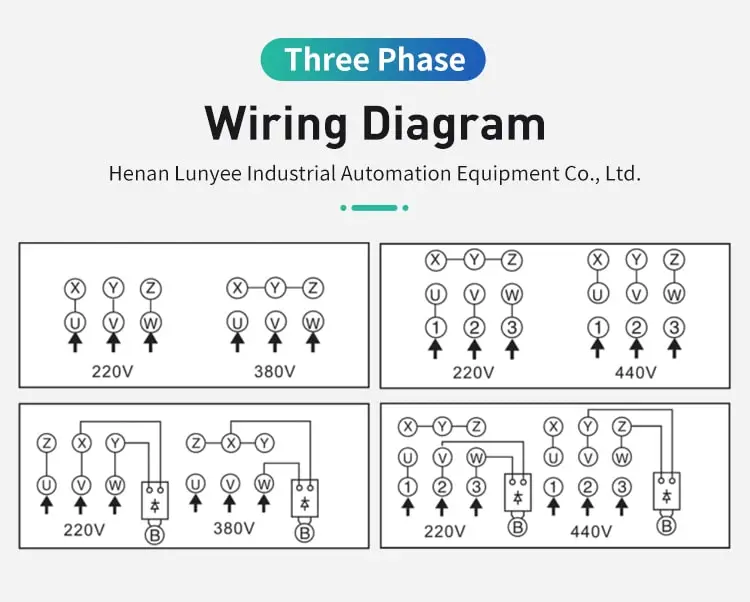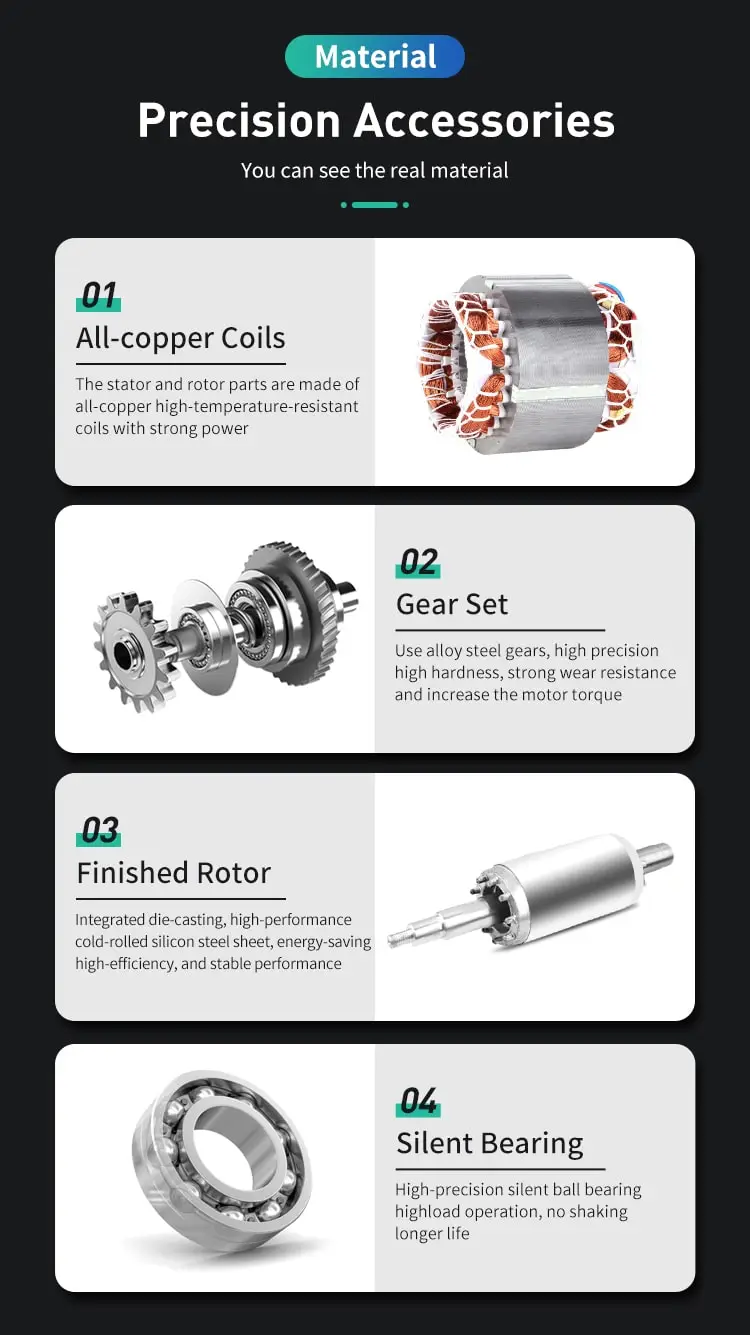By Olivia 2023-01-19 14:06:45

#### How to calculate torque of ac motor?

To begin, torque is a measurement of the rotational force produced by an electric motor. It is a crucial aspect in determining an AC motor's performance since it dictates how much work the motor can accomplish.

To begin, torque is a measure of the rotational force that an electric motor produces. It is an important factor in determining the performance of an AC motor, as it determines how much work the motor can do.

Next, AC motors are electric motors that use alternating current (AC) to generate torque and rotate a shaft. AC motors are widely used in a variety of applications, from small household appliances to large industrial machinery.

To calculate the torque of an AC motor, we can use the following equation:

Torque = (2 * π * Frequency * Power) / (60 * Speed)

where "Frequency" is the frequency of the AC current, "Power" is the power output of the motor, and "Speed" is the rotational speed of the motor's shaft in revolutions per minute (RPM).

There are several factors that can affect the torque of an AC motor , including:

Speed: The speed of the motor's shaft has a direct impact on its torque. As the speed increases, the torque decreases.

Voltage: The voltage supplied to the motor also affects its torque. Increasing the voltage will generally increase the torque, while decreasing the voltage will decrease the torque.

Current: The current flowing through the motor's windings also has an impact on its torque. As the current increases, the torque will also increase.

Number of poles: The number of poles in the motor's stator also affects its torque. A motor with more poles will produce more torque than a motor with fewer poles.

Here are some examples of how to calculate the torque of an AC motor:

Example 1: A 3-phase AC motor with a power output of 5 kW and a speed of 1,500 RPM is operating at a frequency of 50 Hz. What is the torque of the motor?

Using the torque equation above, we can calculate the torque of the motor as follows:

Torque = (2 * π * Frequency * Power) / (60 * Speed)

Torque = (2 * π * 50 * 5,000) / (60 * 1,500)

Torque = 33.5 Nm

Example 2: A single-phase AC motor with a power output of 2 kW and a speed of 1,800 RPM is operating at a frequency of 60 Hz. What is the torque of the motor?

Using the torque equation above, we can calculate the torque of the motor as follows:

Torque = (2 * π * Frequency * Power) / (60 * Speed)

Torque = (2 * π * 60 * 2,000) / (60 * 1,800)

Torque = 11.31 Nm

In conclusion, calculating the torque of an AC motor is an important part of determining its performance. By understanding the factors that affect torque and using the torque equation, we can accurately calculate the torque of an AC motor and ensure that it is operating at its optimal performance.